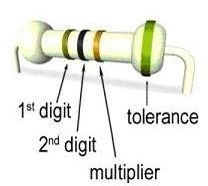# Resistor color code chart and resistance explained for beginners

Page content

## Introduction

Before going into the details of explaining resistor color code charts, I would like to spend a few minutes explaining the basics of resistance for beginners in a simple and clear manner. In the latter sections of this article I have explained the detailed procedure of calculating the resistance of an actual resistor using colour code combination analysis

Resistance of a substance is the property due to which it opposes the flow of electricity through it. Some substances such as metals, acids and salt solutions are very good conductors of electricity. Amongst metals silver, copper and aluminium are very good conductors. This is because of loosely attached electrons in their atoms. When the potential difference is applied these electrons attain a motion and while passing through the atoms of the conductors collided with other electrons generate heat. Whereas some substances such as bakelite, mica, glass and rubber and PVC provide relatively greater hindrance to the passage of electrons through them and are termed as Poor conductors.

Some substances such as paper cotton, mineral oil free from water and acid, ceramic, porcelain and asbestos provide great hindrance to the passage of passage to the electricity as termed as insulators

## Units of Resistance

Unit of resistance is Ohm –A conductor is said to have resistance of one ohm if it allows a current of one Ampere to flow through it when a potential difference of one volt is applied across its ends.

The resistance of a conductor depends upon the following :-

1. Directly proportional to the length of the conductor.
2. Inversely proportional to the area of cross section
3. Nature of material
4. Temperature of conductor

Now the questions is how do you check the resistance of a resistor without having an ohm-meter. Well you just need to figure out the meaning of the color coding printed on the resistors and here is how to go about reading resistor colour codes

## Resistor Colour Codes Explained

The various lines of colour code are allotted a specific value and these are given as follows in this list

1. B-Black 0
2. B –Brown 1
3. R –Red 2
4. O –Orange 3
5. Y – Yellow 4
6. G - Green 5
7. B - Blue 6
8. V – Voilet 7
9. G -GREY 8
10. w - White 9

This has also been shown more colourfully in the adjoining diagram where you can also see the actual colours along with their values

## Calculating Resistance from Codes

The above chart also shows a lot more information than showing the values assigned to each colour. As you can see in this picture of an actual resistor that the coding shows the values on one end and the tolerance at another end which is spaced at a greater distance than normal spacing between the code lines.

Just remember that the 1st stripe is counted as the one which is at the other end than the tolerance strip. You have first value strips and the multiplier strips. so if you see the example of this actual resistor its value comes out to be as calculated belowfirst stripe is brown, then orange, then black

so from the table this comes as 1, 0 and 1000 (multiplier) so the value is

10,000 ohms or 10 K-ohms plus the last line shows the tolerance of the resistor

## 4th Stripe

As explained above the fourth stripe is the measure of tolerance of the resistor which means how much does the resistance change under varying operational conditions such as temperature etc. This is not a great necessity and an average resistor has sufficient tolerance to work satisfactorily in required marine circuits. High level of tolerance is rarely required except in specialized circuits which is beyond the scope of discussion here. If there is no tolerance band present it means that the tolerance is within a standard range of plus-minus 20%